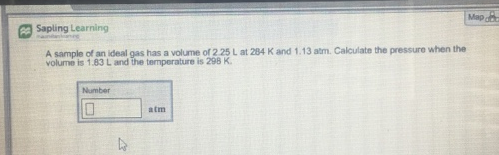# Problem: A sample of an ideal gas has a volume or 2 25 L at 284 K and 1.13 atm. Calculate the pressure when the volume is 1.83 L and the temperature is 298 K

###### FREE Expert Solution###### Problem Details

A sample of an ideal gas has a volume or 2 25 L at 284 K and 1.13 atm. Calculate the pressure when the volume is 1.83 L and the temperature is 298 K